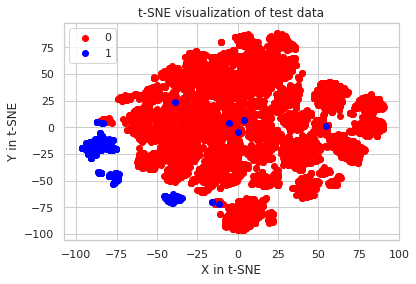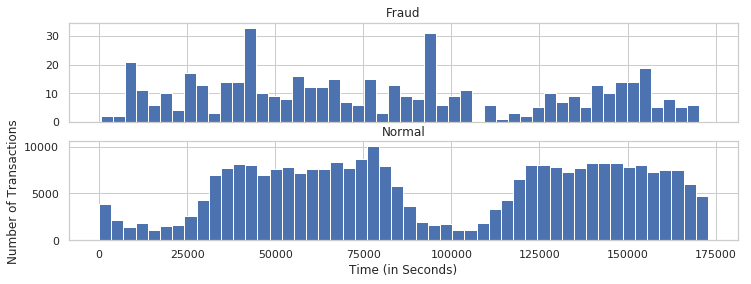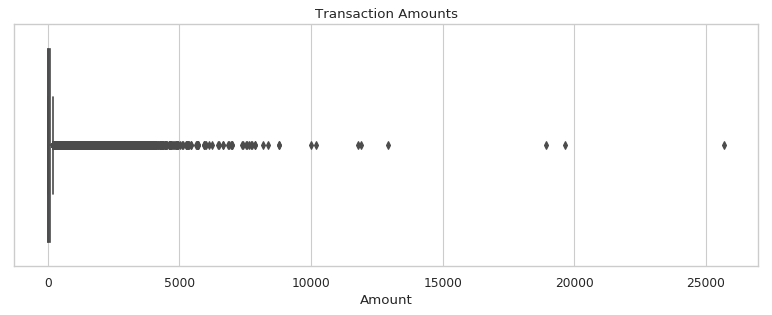How AI Can Support Business IntelligenceIntroduction

Nowadays, AI is rapidly transforming different industries thanks to high data volumes, new advanced algorithms, large data storages, and cloud computing. Business Intelligence is not an exception in this case. BI is one of the top industries where injection an AI solution to a problem can bring immense impact. Small to large companies are using this technology to improve efficiency of business processes and their customer’s experience. AI-powered BI solutions can pull the insights from large datasets, build recommendations based on this data and therefore shape the Business Intelligence decision-making.

For example, recommender systems can suggest a better product to a customer based on his preferences, regression models can predict prices that will find a trade-off between business profit and customer’s paying ability. In this article, we will explore a real-life classification problem of fraud detection.

The goal of this dataset is to predict whether the credit card transaction is fraud. It is important for companies to detect and reject those transactions in real-time. At first, let’s import some libraries that we will be using and load the dataset.

In :
import numpy as np
import scipy as sp
import pandas as pd
import matplotlib as mpl
import matplotlib.pyplot as plt
import seaborn as sns
from sklearn.manifold import TSNE
from sklearn.preprocessing import StandardScaler

# Plotting options
%matplotlib inline
sns.set(style='whitegrid')

As you can see below, there are only numerical features in the dataset:

• Time is describing the number of seconds since the first transaction in the dataset
• Amount – the amount of money transferred
• Class – that’s our label, ‘1’ for fraud transaction other features, ‘0’ for normal
• V_** – those are our main features. They are coded with PCA in order to anonymize sensitive and private data of credit card owners
In :
Out:
In :
transactions.isnull().any().any()
Out:
False

We can see that all dataset is clean and almost ready to be trained with our model.

Exploratory data analysis

For now, let’s explore and visualize dataset a little. At first, we will answer the question, what is the distribution of Class label?

In :
count_classes = pd.value_counts(transactions['Class'], sort = True).sort_index()

print("There are", count_classes.loc, "entries of type '0' in the dataset")
print("There are", count_classes.loc, "entries of type '1' in the dataset")
There are 284315 entries of type '0' in the dataset
There are 492 entries of type '1' in the dataset

So, the dataset is clearly unbalanced. To get a better understanding of the data, we will do some visualizations. Let’s plot our features and see if it is even possible to classify those fraud transactions. In order to visualize multidimensional features, we will use the t-SNE algorithm implemented in Scikit-learn library. That will help us to visualize our dataset in 2-dimensional space. Let’s create a new dataframe that consists of all fraudulent transactions and 10000 normal ones. We suppose that amount will be enough to get some understanding of our features.

In :
df2 = transactions[transactions.Class == 1]
df2 = pd.concat([df2, transactions[transactions.Class == 0].sample(n = 10000)], axis = 0)

Then, we will scale our features. That will help the t-SNE algorithm to get a better understanding of a data and will improve its training speed.

In :
standard_scaler = StandardScaler()
df2_std = standard_scaler.fit_transform(df2.astype(float))

# Set y equal to the target values
y = df2.iloc[:,-1].values

After preprocessing for visualization, we will train out the t-SNE algorithm and finally plot our features in 2-dimensional space.

In :
tsne = TSNE(n_components=2, random_state=0)
x_test_2d = tsne.fit_transform(df2_std)
In :
color_map = {0:'red', 1:'blue'}
plt.figure()
for idx, cl in enumerate(np.unique(y)):
plt.scatter(x = x_test_2d[y==cl,0],
y = x_test_2d[y==cl,1],
c = color_map[idx],
label = cl)
plt.xlabel('X in t-SNE')
plt.ylabel('Y in t-SNE')
plt.legend(loc='upper left')
plt.title('t-SNE visualization of test data')
plt.show()In the plot above we can see that almost all of the fraud labels can be separated from normal ones. Also after this visualization, we can already come up with some ideas of models that probably will and won’t work on this dataset. For example, Linear models such as SVM or Logistic Regression won’t work well on this dataset, because it cannot be split by a simple line. Instead, we can use an ensemble model called Random Forest Classifier. This model will work much better on this problem.

Now, after we understood how all of our data looks like, we will examine separate features. Let’s take a look at the distribution of Time and Amount feature.

In :
f, (ax1, ax2) = plt.subplots(2, 1, sharex=True, figsize=(12,4))

bins = 50

ax1.hist(transactions.Time[transactions.Class == 1], bins = bins)
ax1.set_title('Fraud')

ax2.hist(transactions.Time[transactions.Class == 0], bins = bins)
ax2.set_title('Normal')

plt.xlabel('Time (in Seconds)')
plt.ylabel('Number of Transactions')
plt.show()In :
plt.figure(figsize=(12,4), dpi=80)
sns.boxplot(transactions['Amount'])
plt.title('Transaction Amounts')
Out:
Text(0.5, 1.0, 'Transaction Amounts')From the plots above, we can see that almost all values in the Amount column are below 5000, and most of them are even less than 200. Also, we can’t see any correlation between fraud transactions and Time, so let’s drop this column and we will begin to split our data into train and test datasets.

In :
transactions = transactions.drop(['Time'],axis=1)
X = transactions.drop(labels='Class', axis=1) # Features
y = transactions.loc[:,'Class']               # Label

As the dataset is clearly unbalanced, we will perform OverSampling in order to make ‘0’ and ‘1’ classes equal.

In :
from imblearn.over_sampling import RandomOverSampler
ros = RandomOverSampler(random_state=0)
X_resampled, y_resampled = ros.fit_resample(X, y)
Using TensorFlow backend.

We will use 80% of our data as a training dataset, and 20% as a test.

In :
from sklearn.model_selection import train_test_split

X_train, X_test, y_train, y_test = train_test_split(X_resampled, y_resampled, test_size=0.2, random_state=1)

Model training and evaluation

Let’s import our Random Forest model and fit out training data.

In :
from sklearn.ensemble import RandomForestClassifier
from sklearn.metrics import accuracy_score, f1_score
from sklearn.metrics import confusion_matrix
In :
rf = RandomForestClassifier(n_jobs=-1, random_state=1, n_estimators=20, verbose=0)
In :
rf.fit(X_train, y_train)
Out:
RandomForestClassifier(bootstrap=True, class_weight=None, criterion='gini',
max_depth=None, max_features='auto', max_leaf_nodes=None,
min_impurity_decrease=0.0, min_impurity_split=None,
min_samples_leaf=1, min_samples_split=2,
min_weight_fraction_leaf=0.0, n_estimators=20, n_jobs=-1,
oob_score=False, random_state=1, verbose=0, warm_start=False)

As we have trained our model, let’s see how it performs on the testing set. For our classification metrics, we will use the accuracy score and f1-score. They will show us how efficient our model is on test data.

In :
y_pred = rf.predict(X_test)
print("Accuracy of our model is ", accuracy_score(y_test, y_pred))
print("F1-score metric for our model: ", f1_score(y_test, y_pred))
print(confusion_matrix(y_test,y_pred))
Accuracy of our model is  0.9999736208079067
F1-score metric for our model:  0.9999735922466836
[[56923     3]
[    0 56800]]

As the final result, we get pretty good accuracy.
This means that our classifier can detect a fraud transaction.

Summary

In this article we showed an example of a real-life business problem: detect a fraud card transaction in the dataset. It is very important for the companies to know this information. We were using Random Forest Classifier, and after evaluating our model on test set we got about 99.9% accuracy.

To sum up, this is just one simple problem resolution approach, where injection of AI algorithm can lead to huge impacts for the company. There are a lot of unsolved business real-world problems, but with AI algorithms, computing resources, Big Data and research time almost everything becomes possible to solve.